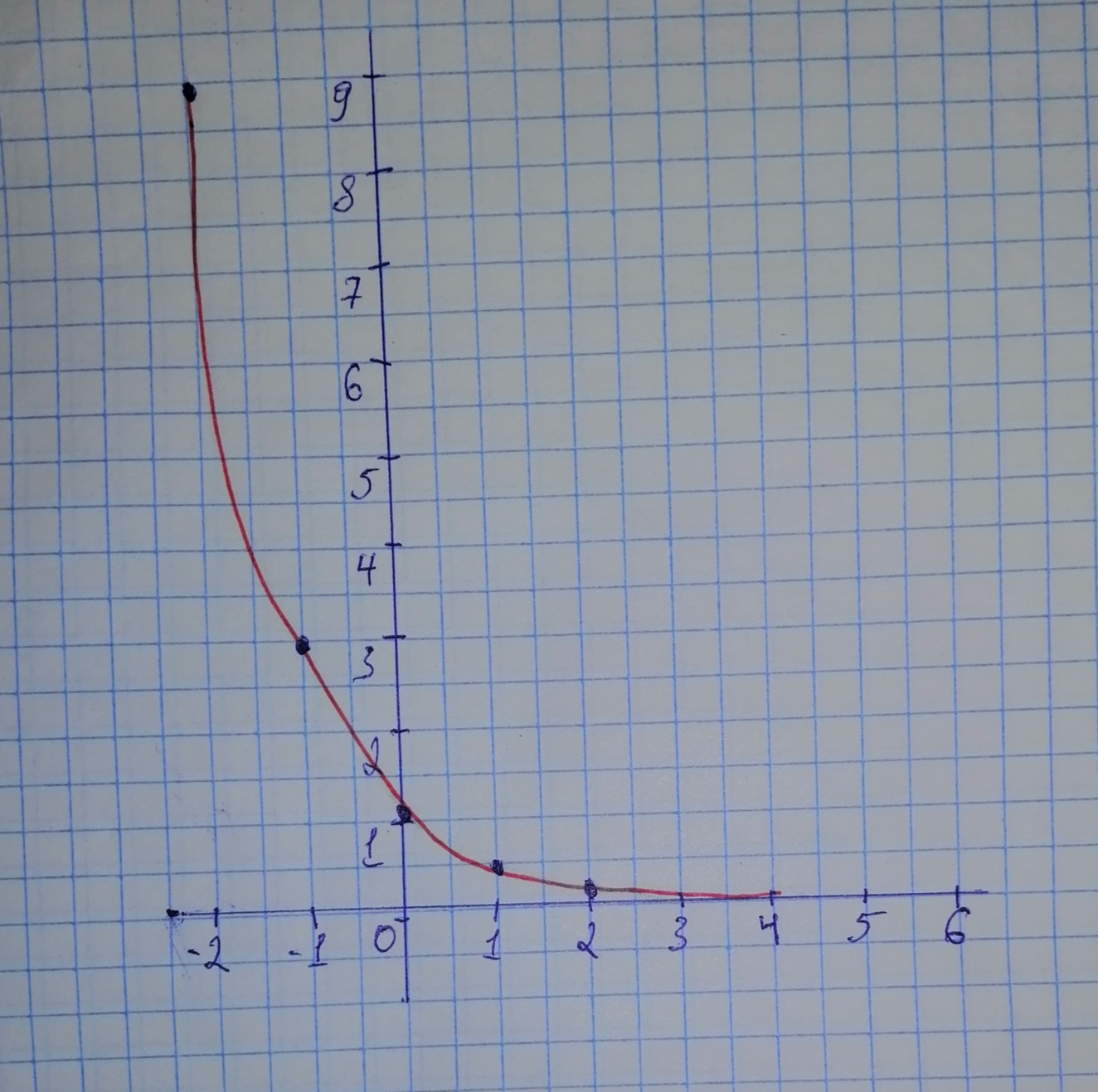# Tell whether the function represents exponential growth or exponential decay. Identify the percent increase or decrease. Then graph the function. f(x)=left(frac{1}{3}right)^{x}Question
Exponential growth and decayTell whether the function represents exponential growth or exponential decay. Identify the percent increase or decrease. Then graph the function.
$$\displaystyle{f{{\left({x}\right)}}}={\left({\frac{{{1}}}{{{3}}}}\right)}^{{{x}}}$$2020-10-24
Step 1
We are given the function:
$$\displaystyle{f{{\left({x}\right)}}}={\left({\frac{{{1}}}{{{3}}}}\right)}^{{{x}}}$$
Step 2
We identify the value of the base. The base, $$\displaystyle{\frac{{{1}}}{{{3}}}},$$ is greater than 0 and smaller than 1, the function represents exponential decay.
Step 3
We make a table of values:
$$\displaystyle{b}{e}{g}\in{\left\lbrace{a}{r}{r}{a}{y}\right\rbrace}{\left\lbrace{\left|{c}\right|}{c}{\mid}\right\rbrace}{h}{l}\in{e}{x}&{y}\backslash{h}{l}\in{e}-{2}&{9}\backslash{h}{l}\in{e}-{1}&{3}\backslash{h}{l}\in{e}{0}&{1}\backslash{h}{l}\in{e}{1}&{\frac{{{1}}}{{{3}}}}\backslash{h}{l}\in{e}{2}&{\frac{{{1}}}{{{9}}}}\backslash{h}{l}\in{e}{e}{n}{d}{\left\lbrace{a}{r}{r}{a}{y}\right\rbrace}$$
Step 4
We plot the points from the table. Then draw a smoth curve from right to left, that begins just above the x-axis, passes throigh the plotted points and moves up to the left.Step 5
The percent of decrease is:
$$\displaystyle{1}\ -\ {\frac{{{1}}}{{{3}}}}={\frac{{{2}}}{{{3}}}}={66}\%$$

### Relevant QuestionsTell whether the function represents exponential growth or exponential decay. Then graph the function.
$$\displaystyle{y}={\left({\frac{{{4}}}{{{3}}}}\right)}^{{{x}}}$$Tell whether the function represents exponential growth or exponential decay. Then graph the function. PSKf(x)=(1.5)^{x}Tell whether the function represents exponential growth or exponential decay. Then graph the function. $$\displaystyle{f{{\left({x}\right)}}}={\left({0.25}\right)}^{{{x}}}$$Determine whether each equation represents exponential growth or exponential decay. Find the rate of increase or decrease for each model. Graph each equation. y=5^xTell whether the function represents exponential growth or exponential decay. Then graph the function.
$$\displaystyle{y}={7}^{{{x}}}$$Tell whether the function represents exponential growth or exponential decay. Then graph the function. $$\displaystyle{y}={3}{e}^{{-{0.75}{x}}}$$$$\displaystyle{y}={80}{\left({0.25}\right)}^{{{x}}}$$The number of teams y remaining in a single elimination tournament can be found using the exponential function $$\displaystyle{y}={128}{\left({\frac{{{1}}}{{{2}}}}\right)}^{{x}}$$ , where x is the number of rounds played in the tournament. a. Determine whether the function represents exponential growth or decay. Explain. b. What does 128 represent in the function? c. What percent of the teams are eliminated after each round? Explain how you know. d. Graph the function. What is a reasonable domain and range for the function? Explain.